# The ENTROPY Procedure (Experimental)

### Simple Regression Analysis

Subsections:

The ENTROPY procedure is similar in syntax to the other regression procedures in SAS. To demonstrate the similarity, suppose the endogenous/dependent variable is y, and x1 and x2 are two exogenous/independent variables of interest. To estimate the parameters in this single equation model using PROC ENTROPY, use the following SAS statements:

   proc entropy;
model y = x1 x2;
run;


#### Test Scores Data Set

Consider the following test score data compiled by Coleman et al. (1966):

title "Test Scores compiled by Coleman et al. (1966)";
data coleman;
input test_score 6.2 teach_sal 6.2 prcnt_prof 8.2
socio_stat 9.2 teach_score 8.2 mom_ed 7.2;
label test_score="Average sixth grade test scores in observed district";
label teach_sal="Average teacher salaries per student (1000s of dollars)";
label prcnt_prof="Percent of students' fathers with professional employment";
label socio_stat="Composite measure of socio-economic status in the district";
label teach_score="Average verbal score for teachers";
label mom_ed="Average level of education (years) of the students' mothers";
datalines;
37.01   3.83   28.87     7.20   26.60   6.19

... more lines ...



This data set contains outliers, and the condition number of the matrix of regressors,, is large, which indicates collinearity among the regressors. Since the maximum entropy estimates are both robust with respect to the outliers and also less sensitive to a high condition number of thematrix, maximum entropy estimation is a good choice for this problem.

To fit a simple linear model to this data by using PROC ENTROPY, use the following statements:

proc entropy data=coleman;
model test_score = teach_sal prcnt_prof socio_stat teach_score mom_ed;
run;


This requests the estimation of a linear model for TEST_SCORE with the following form: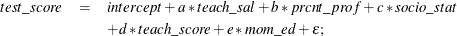This estimation produces the "Model Summary" table in Figure 13.2, which shows the equation variables used in the estimation.

Figure 13.2: Model Summary Table

 Test Scores compiled by Coleman et al. (1966)

The ENTROPY Procedure

Variables(Supports(Weights)) teach_sal prcnt_prof socio_stat teach_score mom_ed Intercept test_score

Since support points and prior weights are not specified in this example, they are not shown in the "Model Summary" table. The next four pieces of information displayed in Figure 13.3 are: the "Data Set Options," the "Minimization Summary," the "Final Information Measures," and the "Observations Processed."

Figure 13.3: Estimation Summary Tables

 Test Scores compiled by Coleman et al. (1966)

The ENTROPY Procedure
GME Estimation Summary

Data Set Options
DATA= WORK.COLEMAN

Minimization Summary
Parameters Estimated 6
Covariance Estimator GME
Entropy Type Shannon
Entropy Form Dual
Numerical Optimizer Quasi Newton

Final Information Measures
Objective Function Value 9.553699
Signal Entropy 9.569484
Noise Entropy -0.01578
Normed Entropy (Signal) 0.990976
Normed Entropy (Noise) 0.999786
Parameter Information Index 0.009024
Error Information Index 0.000214

Observations
Processed
Read 20
Used 20

The item labeled "Objective Function Value" is the value of the entropy estimation criterion for this estimation problem. This measure is analogous to the log-likelihood value in a maximum likelihood estimation. The "Parameter Information Index" and the "Error Information Index" are normalized entropy values that measure the proximity of the solution to the prior or target distributions.

The next table displayed is the ANOVA table, shown in Figure 13.4. This is in the same form as the ANOVA table for the MODEL procedure, since this is also a multivariate procedure.

Figure 13.4: Summary of Residual Errors

GME Summary of Residual Errors
Equation DF Model DF Error SSE MSE Root MSE R-Square Adj RSq
test_score 6 14 175.8 8.7881 2.9645 0.7266 0.6290

The last table displayed is the "Parameter Estimates" table, shown in Figure 13.5. The difference between this parameter estimates table and the parameter estimates table produced by other regression procedures is that the standard error and the probabilities are labeled as approximate.

Figure 13.5: Parameter Estimates

GME Variable Estimates
Variable Estimate Approx Std Err t Value Approx
Pr > |t|
teach_sal 0.287979 0.00551 52.26 <.0001
prcnt_prof 0.02266 0.00323 7.01 <.0001
socio_stat 0.199777 0.0308 6.48 <.0001
teach_score 0.497137 0.0180 27.61 <.0001
mom_ed 1.644472 0.0921 17.85 <.0001
Intercept 10.5021 0.3958 26.53 <.0001

The parameter estimates produced by the REG procedure for this same model are shown in Figure 13.6. Note that the parameters and standard errors from PROC REG are much different than estimates produced by PROC ENTROPY.

symbol v=dot h=1 c=green;

proc reg data=coleman;
model test_score = teach_sal prcnt_prof socio_stat teach_score mom_ed;
plot rstudent.*obs.
/ vref= -1.714 1.714 cvref=blue lvref=1
HREF=0 to 30 by 5 cHREF=red  cframe=ligr;
run;


Figure 13.6: REG Procedure Parameter Estimates

 Test Scores compiled by Coleman et al. (1966)

The REG Procedure
Model: MODEL1
Dependent Variable: test_score

Parameter Estimates
Variable DF Parameter
Estimate
Standard
Error
t Value Pr > |t|
Intercept 1 19.94857 13.62755 1.46 0.1653
teach_sal 1 -1.79333 1.23340 -1.45 0.1680
prcnt_prof 1 0.04360 0.05326 0.82 0.4267
socio_stat 1 0.55576 0.09296 5.98 <.0001
teach_score 1 1.11017 0.43377 2.56 0.0227
mom_ed 1 -1.81092 2.02739 -0.89 0.3868

This data set contains two outliers, observations 3 and 18. These can be seen in a plot of the residuals shown in Figure 13.7

Figure 13.7: PROC REG Residuals with Outliers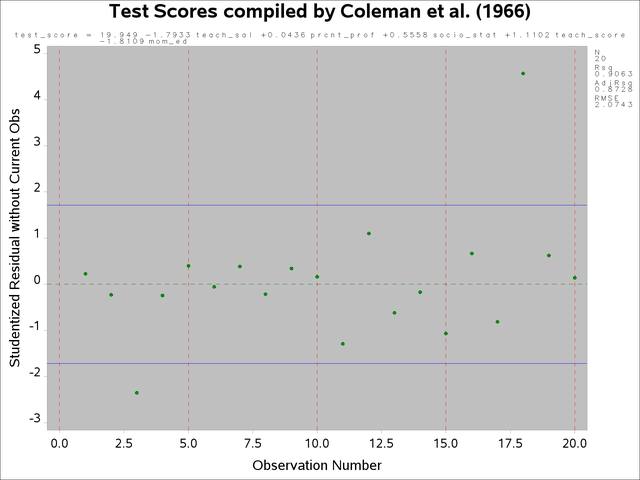The presence of outliers suggests that a robust estimator such as M-estimator in the ROBUSTREG procedure should be used. The following statements use the ROBUSTREG procedure to estimate the model.

proc robustreg data=coleman;
model test_score = teach_sal prcnt_prof
socio_stat teach_score mom_ed;
run;


The results of the estimation are shown in Figure 13.8.

Figure 13.8: M-Estimation Results

 Test Scores compiled by Coleman et al. (1966)

The ROBUSTREG Procedure

Parameter Estimates
Parameter DF Estimate Standard
Error
95% Confidence Limits Chi-Square Pr > ChiSq
Intercept 1 29.3416 6.0381 17.5072 41.1761 23.61 <.0001
teach_sal 1 -1.6329 0.5465 -2.7040 -0.5618 8.93 0.0028
prcnt_prof 1 0.0823 0.0236 0.0361 0.1286 12.17 0.0005
socio_stat 1 0.6653 0.0412 0.5846 0.7461 260.95 <.0001
teach_score 1 1.1744 0.1922 0.7977 1.5510 37.34 <.0001
mom_ed 1 -3.9706 0.8983 -5.7312 -2.2100 19.54 <.0001
Scale 1 0.6966

Note that TEACH_SAL(VAR1) and MOM_ED(VAR5) change greatly when the robust estimation is used. Unfortunately, these two coefficients are negative, which implies that the test scores increase with decreasing teacher salaries and decreasing levels of the mother’s education. Since ROBUSTREG is robust to outliers, they are not causing the counterintuitive parameter estimates.

The condition number of the regressor matrixalso plays a important role in parameter estimation. The condition number of the matrix can be obtained by specifying the COLLIN option in the PROC ENTROPY statement.

proc entropy data=coleman collin;
model test_score = teach_sal prcnt_prof socio_stat teach_score mom_ed;
run;


The output produced by the COLLIN option is shown in Figure 13.9.

Figure 13.9: Collinearity Diagnostics

 Test Scores compiled by Coleman et al. (1966)

The ENTROPY Procedure

Collinearity Diagnostics
Number Eigenvalue Condition
Number
Proportion of Variation
teach_sal prcnt_prof socio_stat teach_score mom_ed Intercept
1 4.978128 1.0000 0.0007 0.0012 0.0026 0.0001 0.0001 0.0000
2 0.937758 2.3040 0.0006 0.0028 0.2131 0.0001 0.0000 0.0001
3 0.066023 8.6833 0.0202 0.3529 0.6159 0.0011 0.0000 0.0003
4 0.016036 17.6191 0.7961 0.0317 0.0534 0.0059 0.0083 0.0099
5 0.001364 60.4112 0.1619 0.3242 0.0053 0.7987 0.3309 0.0282
6 0.000691 84.8501 0.0205 0.2874 0.1096 0.1942 0.6607 0.9614

The condition number of thematrix is reported to be 84.85. This means that the condition number ofis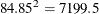, which is very large.

Ridge regression can be used to offset some of the problems associated with ill-conditionedmatrices. Using the formula for the ridge value as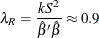where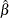and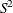are the least squares estimators ofandand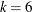. A ridge regression of the test score model was performed by using the data set with the outliers removed. The following PROC REG code performs the ridge regression:

data coleman;
set coleman;
if _n_ = 3 or _n_ = 18 then delete;
run;

proc reg data=coleman ridge=0.9 outest=t noprint;
model test_score = teach_sal prcnt_prof socio_stat teach_score mom_ed;
run;

proc print data=t;
run;


The results of the estimation are shown in Figure 13.10.

Figure 13.10: Ridge Regression Estimates

 Test Scores compiled by Coleman et al. (1966)

Obs _MODEL_ _TYPE_ _DEPVAR_ _RIDGE_ _PCOMIT_ _RMSE_ Intercept teach_sal prcnt_prof socio_stat teach_score mom_ed test_score
1 MODEL1 PARMS test_score . . 0.78236 29.7577 -1.69854 0.085118 0.66617 1.18400 -4.06675 -1
2 MODEL1 RIDGE test_score 0.9 . 3.19679 9.6698 -0.08892 0.041889 0.23223 0.60041 1.32168 -1

Note that the ridge regression estimates are much closer to the estimates produced by the ENTROPY procedure that uses the original data set. Ridge regressions are not robust to outliers as maximum entropy estimates are. This might explain why the estimates still differ for TEACH_SAL.